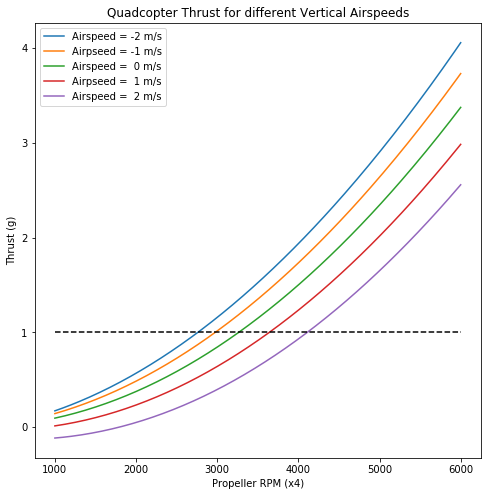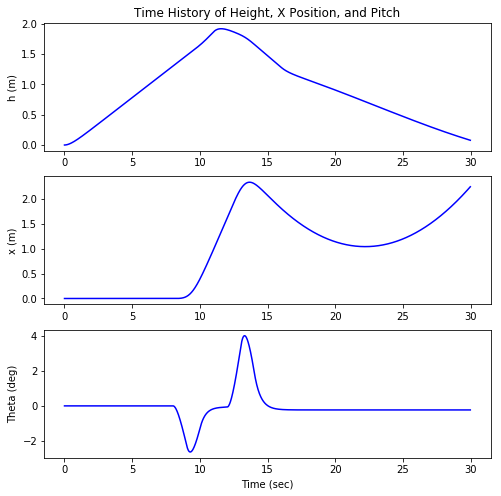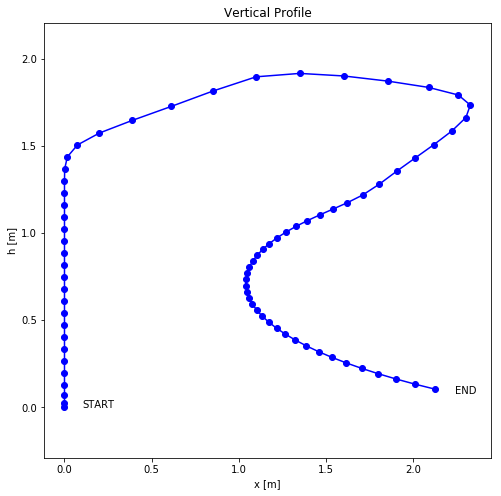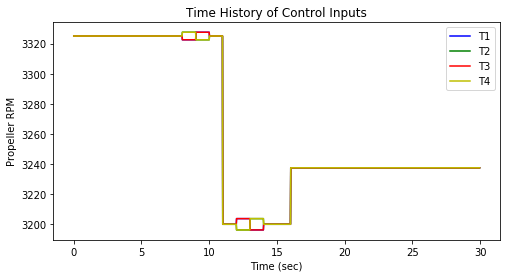# Modeling Vehicle Dynamics – 6DOF Nonlinear Simulation

Here we take all the equations of motion we have derived and numerically integrate them to generate a simulation of the vehicle motion and dynamics.

This post is the 3rd in a series on modeling and simulation of a quadcopter’s vehicle dynamics. The previous posts can be found here:

1. Modeling Vehicle Dynamics – Euler Angles
2. Modeling Vehicle Dynamics – Quadcopter Equations of Motion
3. Modeling Vehicle Dynamics – 6DOF Nonlinear Simulation

## Visualization

Below is a javascript visualization of the trajectory plotted in the below Jupyter Notebook.

Use left click to rotate, middle click to zoom, and right click to pan (when camera is not locked)

## Programming a Simulation

I have created the simulation in a Jupyter notebook which uses Python code. You can download a copy of the notebook to make changes and try running it from my GitHub here:

### Example Control Inputs:

In order for the simulation to show something interesting without any control laws in place, I have hard coded in a small set of propeller commands:

• For time = 0.0 – 10.0 seconds:  Apply positive climb acceleration
• For time = 8.0 – 9.0 seconds:  Apply forward pitch rate
• For time = 9.0 – 10.0 seconds:  Remove forward pitch rate
• For time = 12.0 – 13.0 seconds:  Apply negative pitch rate
• For time = 13.0 – 14.0 seconds:  Remove negative pitch rate
• For time = 16.0 and after: Apply slight positive climb acceleration

After the simulation runs you can see the plots at the bottom of the notebook that show the effect these control inputs produce.

A nonlinear 6 degree-of-freedom simulation of a quadcopter.

The following are some of the assumptions made in this simulation:

• Rigid body dynamics
• Products of inertia are all zero due to symmetry of vehicle
• Simplified rotor aerodynamics based on actuator disk theory
• Assumes rigid propellers
• 1-D, incompressible flow
• Uniform airflow over disk representing area of propeller travel
• No downwash or airflow interference from nearby structure
• Angle of attack is assumed to be within linear lift coefficient region
• Small angle approximation applied for angle of attack
• Propeller blade angle linearly twists from root to tip
• No delays or motor dynamics are included, motor commands achieve desired RPM instantaneously

### Simulation Settings¶

This first cell will hold the user defined parameters:

• Configuration and weights of the quadcopter
• Simulation time settings
• Initial conditions
In :
import numpy as np
import matplotlib.pyplot as plt
# Visualizations will be shown in the notebook.
%matplotlib inline

# Physical Constants
m = 0.1         #kg
Ixx = 0.00062   #kg-m^2
Iyy = 0.00113   #kg-m^2
Izz = 0.9*(Ixx + Iyy) #kg-m^2 (Assume nearly flat object, z=0)
dx = 0.114      #m
dy = 0.0825     #m
g = 9.81  #m/s/s
DTR = 1/57.3; RTD = 57.3

# Simulation time and model parameters
tstep = 0.02            # Sampling time (sec)
simulation_time = 30   # Length of time to run simulation (sec)
t = np.arange(0,simulation_time,tstep)   # time array

# Model size
n_states = 12  # Number of states
n_inputs = 4   # Number of inputs

# Initialize State Conditions
x = np.zeros((n_states,np.size(t)))  # time history of state vectors
# Initial height
x[11,0] = 0.0

# Initialize inputs
u = np.zeros((n_inputs,np.size(t)))  # time history of input vectors
# Initial control inputs
u[:,0] = np.zeros(4)


## Aerodynamics Model¶

### Propeller Thrust¶

Using actuator disk theory, which assumes airflow is constant throughout the disk representing the travel of the propeller blades.

Calculate induced velocity ($v_i$) and thrust (T) by solving the system of 3 equations:

Local airstream velocity at center of propeller$V' = \sqrt{ U^2 + V^2 + (W - v_i)^2}$

Total thrust of propeller using local angle of attack where propeller twist is assumed to be linear from root to tip, and averaged over one revolution:$T = \frac{1}{4}\rho abcR [(W - v_i) \Omega R + \frac{2}{3}(\Omega R)^2(\theta_0+\frac{3}{4}\theta_1) + (U^2+V^2)(\theta_0 + \frac{1}{2}\theta_1) ]$

Thrust equals the change in velocity times the mass flow rate$T = \eta 2v_i\rho A V'$

where:

[U,V,W] = Longitudinal, lateral, and vertical components of local airstream velocity$v_i$ = induced velocity generated by propeller
A = area of disk$\rho$ = density of air
a = lift curve slope$(\frac{dC_L}{d\alpha})$
b = number of propeller blades
c = mean chord length of propeller blades$\Omega$ = Angular rate of propeller rotation$\theta_0$ = propeller pitch angle at root$\theta_1$ = delta pitch twist at propeller tip (assumes linear twist along blade)$\eta$ = effeciency of propeller (value of 0-1)

### Propeller Normal Force¶

Propellers will also produce a normal force, perpendicular to the thrust force. This is not currently modeled.

### Gyroscopic Force¶

The yawing moment from the propellers will come from the gyroscopic force of the rotating motors and propellers. This is not currently modeled.

Reference: Stevens, B., Lewis, F., and Johnson, E., Aircraft Control and Simulation: Dynamics, Controls Design, and Autonomous Systems. 3rd ed., John Wiley & Sons, 2016.
(Note: topic not covered in previous editions)

In :
from scipy.optimize import fsolve

# Propeller Thrust equations as a function of propeller induced velocity, vi
def thrustEqn(vi, *prop_params):

# Unpack parameters
R,A,rho,a,b,c,eta,theta0,theta1,U,V,W,Omega = prop_params

# Calculate local airflow velocity at propeller with vi, V'
Vprime = np.sqrt(U**2 + V**2 + (W - vi)**2)

# Calculate Thrust averaged over one revolution of propeller using vi
Thrust = 1/4 * rho * a * b * c * R * \
( (W - vi) * Omega * R + 2/3 * (Omega * R)**2 * (theta0 + 3/4 * theta1) + \
(U**2 + V**2) * (theta0 + 1/2 * theta1) )

# Calculate residual for equation: Thrust = mass flow rate * delta Velocity
residual = eta * 2 * vi * rho * A * Vprime - Thrust

return residual

def Fthrust(x, u, dx, dy):
# Inputs: Current state x[k], Commanded Propeller RPM inputs u[k],
#         Propeller location distances dx, dy (m)
# Returns: Thrust vector for 4 propellers (Newtons)

# Propeller Configuration parameters

R = 0.0762   # propeller length/ disk radius (m)
A = np.pi * R ** 2
rho = 1.225  #kg/m^3  at MSL
a = 5.7      # Lift curve slope used in example in Stevens & Lewis
b = 2        # number of blades
c = 0.0274   # mean chord length (m)
eta = 1      # propeller efficiency

# Manufacturer propeller length x pitch specification:
p_diameter = 6  #inches
p_pitch = 3   #inches

theta0 = 2*np.arctan2(p_pitch, (2 * np.pi * 3/4 * p_diameter/2))
theta1 = -4 / 3 * np.arctan2(p_pitch, 2 * np.pi * 3/4 * p_diameter/2)

# Local velocity at propeller from vehicle state information
ub, vb, wb = x, x, x
p, q, r = x, x, x
# Transofrm velocity to local propeller location:
#     [U,V,W] = [ub,vb,wb] + [p,q,r] x [dx,dy,0]
U = ub - r * dy
V = vb + r * dx
W = wb - q * dx + p * dy

# Convert commanded RPM to rad/s
Omega = 2 * np.pi / 60 * u

#Collect propeller config, state, and input parameters
prop_params = (R,A,rho,a,b,c,eta,theta0,theta1,U,V,W,Omega)

# Numerically solve for propeller induced velocity, vi
# using nonlinear root finder, fsolve, and prop_params
vi0 = 0.1    # initial guess for vi
vi = fsolve(thrustEqn, vi0, args=prop_params)

# Plug vi back into Thrust equation to solve for T
Vprime = np.sqrt(U**2 + V**2 + (W - vi)**2)
Thrust = eta * 2 * vi * rho * A * Vprime

return Thrust

# Torque function
def T(F,dx,dy):
# Returns torque about cg given thrust force and dx,dy distance from cg

#### PLACEHOLDER ####
return 0

# Plot Thrust as a function of RPM for various vertical velocity conditions
RPM = np.linspace(1000,6000,200)
vertvel = np.array([0,0,1] + 9*)
Thrust_m2vel = np.array([Fthrust(2*vertvel,rpmIn,dx,dy) for rpmIn in RPM])
Thrust_m1vel = np.array([Fthrust(1*vertvel,rpmIn,dx,dy) for rpmIn in RPM])
Thrust_0vel  = np.array([Fthrust(0*vertvel,rpmIn,dx,dy) for rpmIn in RPM])
Thrust_p1vel = np.array([Fthrust(-1*vertvel,rpmIn,dx,dy) for rpmIn in RPM])
Thrust_p2vel = np.array([Fthrust(-2*vertvel,rpmIn,dx,dy) for rpmIn in RPM])
fig = plt.figure(figsize=(8,8))
plt.plot(RPM, 4 * Thrust_m2vel / (m*g) )
plt.plot(RPM, 4 * Thrust_m1vel / (m*g) )
plt.plot(RPM, 4 * Thrust_0vel / (m*g) )
plt.plot(RPM, 4 * Thrust_p1vel / (m*g) )
plt.plot(RPM, 4 * Thrust_p2vel / (m*g) )
plt.plot(RPM, np.ones(np.size(RPM)), 'k--')
plt.legend(('Airspeed = -2 m/s','Airpseed = -1 m/s','Airspeed =  0 m/s', \
'Airpseed =  1 m/s','Airspeed =  2 m/s'), loc='upper left')
plt.xlabel('Propeller RPM (x4)')
plt.ylabel('Thrust (g)')
plt.title('Quadcopter Thrust for different Vertical Airspeeds')
plt.show()## Equations of Motion¶

Calculate the derivative of the state as a function of the current state and control inputs$\dot{u} = -g\sin(\theta) + rv - qw$$\dot{v} = g\sin(\phi)\cos(\theta) -ru + pw$$\dot{w} = \frac{1}{m}(-F_{z}) + g\cos(\phi)\cos(\theta) + qu - pv$$\dot{p} = \frac{1}{I_{xx}}(L + (I_{yy}-I_{zz})qr)$$\dot{q} = \frac{1}{I_{yy}}(M + (I_{zz}-I_{xx})pr)$$\dot{r} = \frac{1}{I_{zz}}(N + (I_{xx}-I_{yy})pq)$$\dot{\phi} = p + (q \sin\phi + r \cos\phi) \tan\theta$$\dot{\theta} = q \cos\phi - r \sin\phi$$\dot{\psi} = (q \sin\phi + r \cos\phi) \sec\theta$$\dot{x}^{E} = c_{\theta}c_{\psi}u^{b} + (-c_{\phi}s_{\psi}+s_{\phi}s_{\theta}c_{\psi})v^{b} + (s_{\phi}s_{\psi}+c_{\phi}s_{\theta}c_{\psi})w^{b}$$\dot{y}^{E} = c_{\theta}s_{\psi}u^{b} + (c_{\phi}c_{\psi}+s_{\phi}s_{\theta}s_{\psi})v^{b} + (-s_{\phi}c_{\psi}+c_{\phi}s_{\theta}s_{\psi})w^{b}$$\dot{h}^{E} = -1*(-s_{\theta}u^{b} + s_{\phi}c_{\theta}v^{b} + c_{\phi}c_{\theta}w^{b})$

where$F_{z} = F_1 + F_2 + F_3 + F_4$$L = F_1 d_{1y} - F_2 d_{2y} - F_3 d_{3y} + F_4 d_{4y}$$M = -F_1 d_{1x} + F_2 d_{2x} - F_3 d_{3x} + F_4 d_{4x}$$N = -T(F_1,d_{1x},d_{1y}) - T(F_2,d_{2x},d_{2y}) + T(F_3,d_{3x},d_{3y}) + T(F_4,d_{4x},d_{4y})$

and$h^E$ is positive in the up direction.

In :
# Nonlinear Dynamics Equations of Motion
def stateDerivative(x,u):
# Inputs: state vector (x), input vector (u)
# Returns: time derivative of state vector (xdot)

#  State Vector Reference:
#idx  0, 1, 2, 3, 4, 5,  6,   7,   8,   9, 10, 11
#x = [u, v, w, p, q, r, phi, the, psi, xE, yE, hE]

# Store state variables in a readable format
ub = x
vb = x
wb = x
p = x
q = x
r = x
phi = x
theta = x
psi = x
xE = x
yE = x
hE = x

# Calculate forces from propeller inputs (u)
F1 = Fthrust(x, u,  dx,  dy)
F2 = Fthrust(x, u, -dx, -dy)
F3 = Fthrust(x, u,  dx, -dy)
F4 = Fthrust(x, u, -dx,  dy)
Fz = F1 + F2 + F3 + F4
L = (F2 + F3) * dy - (F1 + F4) * dy
M = (F1 + F3) * dx - (F2 + F4) * dx
N = -T(F1,dx,dy) - T(F2,dx,dy) + T(F3,dx,dy) + T(F4,dx,dy)

# Pre-calculate trig values
cphi = np.cos(phi);   sphi = np.sin(phi)
cthe = np.cos(theta); sthe = np.sin(theta)
cpsi = np.cos(psi);   spsi = np.sin(psi)

# Calculate the derivative of the state matrix using EOM
xdot = np.zeros(12)

xdot = -g * sthe + r * vb - q * wb  # = udot
xdot = g * sphi*cthe - r * ub + p * wb # = vdot
xdot = 1/m * (-Fz) + g*cphi*cthe + q * ub - p * vb # = wdot
xdot = 1/Ixx * (L + (Iyy - Izz) * q * r)  # = pdot
xdot = 1/Iyy * (M + (Izz - Ixx) * p * r)  # = qdot
xdot = 1/Izz * (N + (Ixx - Iyy) * p * q)  # = rdot
xdot = p + (q*sphi + r*cphi) * sthe / cthe  # = phidot
xdot = q * cphi - r * sphi  # = thetadot
xdot = (q * sphi + r * cphi) / cthe  # = psidot

xdot = cthe*cpsi*ub + (-cphi*spsi + sphi*sthe*cpsi) * vb + \
(sphi*spsi+cphi*sthe*cpsi) * wb  # = xEdot

xdot = cthe*spsi * ub + (cphi*cpsi+sphi*sthe*spsi) * vb + \
(-sphi*cpsi+cphi*sthe*spsi) * wb # = yEdot

xdot = -1*(-sthe * ub + sphi*cthe * vb + cphi*cthe * wb) # = hEdot

return xdot


## Control Law¶

This is a placeholder for where the control law will determine the input commands it sends to the propeller motors.

For now, we can try hard coding in some example open loop control inputs at different times to see how the model dynamics respond.

In :
def controlInputs(x, t):
# Inputs: Current state x[k], time t
# Returns: Control inputs u[k]

#### Placeholder Function ####

# Trim RPM for all 4 propellers to provide thrust for a level hover
trim = 3200

pitch_cmd = 0
roll_cmd = 0
climb_cmd = 0
yaw_cmd = 0

# Example open loop control inputs to test dynamics:
#  Climb
if t < 11.0:
climb_cmd = 500

#  Pitch Forward
if t > 8.0:
pitch_cmd = -10
if t > 9.0:
pitch_cmd = 10
if t > 10.0:
pitch_cmd = 0

#  Pitch Backward
if t > 12.0:
pitch_cmd = 15
if t > 13.0:
pitch_cmd = -15
if t > 14.0:
pitch_cmd = 0

#  Increase lift
if t > 16.0:
climb_cmd = 150

# RPM command based on pitch, roll, climb, yaw commands
u = np.zeros(4)
u = trim + ( pitch_cmd + roll_cmd + climb_cmd - yaw_cmd) / 4
u = trim + (-pitch_cmd - roll_cmd + climb_cmd - yaw_cmd) / 4
u = trim + ( pitch_cmd - roll_cmd + climb_cmd + yaw_cmd) / 4
u = trim + (-pitch_cmd + roll_cmd + climb_cmd + yaw_cmd) / 4

return u


## Numerical Integration¶

In :
# 4th Order Runge Kutta Calculation
def RK4(x,u,dt):
# Inputs: x[k], u[k], dt (time step, seconds)
# Returns: x[k+1]

# Calculate slope estimates
K1 = stateDerivative(x, u)
K2 = stateDerivative(x + K1 * dt / 2, u)
K3 = stateDerivative(x + K2 * dt / 2, u)
K4 = stateDerivative(x + K3 * dt, u)

# Calculate x[k+1] estimate using combination of slope estimates
x_next = x + 1/6 * (K1 + 2*K2 + 2*K3 + K4) * dt

return x_next

# March through time array and numerically solve for vehicle states

for k in range(0, np.size(t) - 1):

# Determine control inputs based on current state
u[:,k] = controlInputs(x[:,k], t[k])

# Predict state after one time step
x[:,k+1] = RK4(x[:,k], u[:,k], tstep)



## Plot Results¶

In :
plt.figure(1, figsize=(8,8))
plt.subplot(311)
plt.plot(t,x[11,:],'b',label='h')
plt.ylabel('h (m)')
#plt.xlabel('Time (sec)')
#plt.legend(loc='best')
plt.title('Time History of Height, X Position, and Pitch')

plt.subplot(312)
plt.plot(t,x[9,:],'b',label='x')
plt.ylabel('x (m)')
#plt.xlabel('Time (sec)')

plt.subplot(313)
plt.plot(t,x[7,:]*RTD,'b',label='theta')
plt.ylabel('Theta (deg)')
plt.xlabel('Time (sec)')

plt.figure(2, figsize=(8,8))
ax = plt.subplot(1,1,1)
plt.plot(x[9,0:-1:20],x[11,0:-1:20],'bo-',label='y')
plt.text(x[9,0] + 0.1, x[11,0],'START')
plt.text(x[9,-1], x[11,-1],'END')
plt.ylabel('h [m]'); plt.xlabel('x [m]')
ax.axis('equal')
#plt.legend(loc='best')
plt.title('Vertical Profile')

plt.figure(3, figsize=(8,4))
plt.plot(t[0:-1],u[0,0:-1],'b',label='T1')
plt.plot(t[0:-1],u[1,0:-1],'g',label='T2')
plt.plot(t[0:-1],u[2,0:-1],'r',label='T3')
plt.plot(t[0:-1],u[3,0:-1],'y',label='T4')
plt.xlabel('Time (sec)')
plt.ylabel('Propeller RPM')
plt.legend(loc='best')
plt.title('Time History of Control Inputs')

plt.show()In :
#Store time, input, and state data to a json file for visualization
import json, codecs
log_filename = "visualizer/sim_log.json"
with open(log_filename, "w") as logfile:
logfile.write("var sim_data = ")
json.dump([t.tolist(), u.tolist(),x.tolist()], \
codecs.open(log_filename, 'a', encoding='utf-8'), \
separators=(',', ':'), indent=4)


## 3 thoughts on “Modeling Vehicle Dynamics – 6DOF Nonlinear Simulation”

1. Hi Charles,

I am interested in understanding the derivation of the Euler equations of motion that you have used in your simulation. I am familiar with these equations but have them in a different form (I am a naval architect and have them in a ship dynamics context) and so am trying to reconcile the two forms. Do you have a particular reference for the equations you have used?

cheers

Richard

2. Hi there,
I was just wondering what would be the expression that would be used to calculate Torque. I mean the the below code returns a value of zero isn’t it?

def T(F,dx,dy):
# Returns torque about cg given thrust force and dx,dy distance from cg

#### PLACEHOLDER ####
return 0

I have been going through various sources to finalize on the expression. There are sources which gives expressions such as Torque = Cq * Omega^2 , where Cq is the torque coefficient and Omega the RPM (http://www.cs.cmu.edu/afs/cs.cmu.edu/academic/class/16311/www/s15/syllabus/ppp/Lec08-Control3.pdf) . I am bit confused there as the expression doesn’t contian information regards to the distance from CoM.

1. Hello,

Faced same issue.
Found the answer in the text:

“Propeller Normal Force¶
Propellers will also produce a normal force, perpendicular to the thrust force. This is not currently modeled.

Gyroscopic Force¶
The yawing moment from the propellers will come from the gyroscopic force of the rotating motors and propellers. This is not currently modeled.”

Besides, tried to simplify the model for thrust calculation by using C*Omega^2. Behavior is very different and not satisfactory.